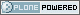# Neuroimaging Informatics Technology Initiative

##### Personal tools
You are here: Home » intent_code.html

# intent_code.html

## intent_code

```
/---------------------------------------------------------------------------/
/* INTERPRETATION OF VOXEL DATA:
----------------------------
The intent_code field can be used to indicate that the voxel data has
some particular meaning.  In particular, a large number of codes is
given to indicate that the the voxel data should be interpreted as
being drawn from a given probability distribution.   VECTOR-VALUED DATASETS:
----------------------
The 5th dimension of the dataset, if present (i.e., dim=5 and
dim > 1), contains multiple values (e.g., a vector) to be stored
at each spatiotemporal location.  For example, the header values
- dim = 5
- dim = 64
- dim = 64
- dim = 20
- dim = 1     (indicates no time axis)
- dim = 3
- datatype = DT_FLOAT
- intent_code = NIFTI_INTENT_VECTOR
mean that this dataset should be interpreted as a 3D volume (64x64x20),
with a 3-vector of floats defined at each point in the 3D grid.
A program reading a dataset with a 5th dimension may want to reformat
the image data to store each voxels' set of values together in a struct
or array.  This programming detail, however, is beyond the scope of the
NIFTI-1 file specification!  Uses of dimensions 6 and 7 are also not
specified here.
STATISTICAL PARAMETRIC DATASETS (i.e., SPMs):
--------------------------------------------
Values of intent_code from NIFTI_FIRST_STATCODE to NIFTI_LAST_STATCODE
(inclusive) indicate that the numbers in the dataset should be interpreted
as being drawn from a given distribution.  Most such distributions have
auxiliary parameters (e.g., NIFTI_INTENT_TTEST has 1 DOF parameter).
If the dataset DOES NOT have a 5th dimension, then the auxiliary parameters
are the same for each voxel, and are given in header fields intent_p1,
intent_p2, and intent_p3.
If the dataset DOES have a 5th dimension, then the auxiliary parameters
are different for each voxel.  For example, the header values
- dim = 5
- dim = 128
- dim = 128
- dim = 1      (indicates a single slice)
- dim = 1      (indicates no time axis)
- dim = 2
- datatype = DT_FLOAT
- intent_code = NIFTI_INTENT_TTEST
mean that this is a 2D dataset (128x128) of t-statistics, with the
t-statistic being in the first "plane" of data and the degrees-of-freedom
parameter being in the second "plane" of data.
If the dataset 5th dimension is used to store the voxel-wise statistical
parameters, then dim must be 1 plus the number of parameters required
by that distribution (e.g., intent_code=NIFTI_INTENT_TTEST implies dim
must be 2, as in the example just above).
Note: intent_code values 2..10 are compatible with AFNI 1.5x (which is
why there is no code with value=1, which is obsolescent in AFNI).
OTHER INTENTIONS:
----------------
The purpose of the intent_* fields is to help interpret the values
stored in the dataset.  Some non-statistical values for intent_code
and conventions are provided for storing other complex data types.
The intent_name field provides space for a 15 character (plus 0 byte)
`name` string for the type of data stored. Examples:
- intent_code = NIFTI_INTENT_ESTIMATE; intent_name = "T1";
could be used to signify that the voxel values are estimates of the
NMR parameter T1.
- intent_code = NIFTI_INTENT_TTEST; intent_name = "House";
could be used to signify that the voxel values are t-statistics
for the significance of `activation` response to a House stimulus.
- intent_code = NIFTI_INTENT_DISPVECT; intent_name = "ToMNI152";
could be used to signify that the voxel values are a displacement
vector that transforms each voxel (x,y,z) location to the
corresponding location in the MNI152 standard brain.
- intent_code = NIFTI_INTENT_SYMMATRIX; intent_name = "DTI";
could be used to signify that the voxel values comprise a diffusion
tensor image.
If no data name is implied or needed, intent_name should be set to 0.

```

### DEFINED CODES

nifti1 intent codes

Created by Alex Clark